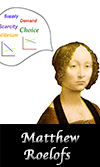Center for Instructional
Innovation and Assessment

# INNOVATIVE TEACHING SHOWCASE

2000
2001
Thor Hansen
Tim Pilgrim
Matthew Roelofs
Linda Smeins
Goals Contents
GOALSMatthew Roelofs
Department of Economics

## Institutional Goals

Listed below are selected learning outcomes in the area of critical thinking that Western Washington University is actively integrating into its curriculum. Each learning outcome is listed with its definition, along with a description of how Matthew Roelofs' teaching strategies meet each of these student learning outcome goals.

### Quantitative Reasoning

Learning Outcomes Definition Course Outcomes
Mathematical Information Represent mathematical information symbolically, visually, numerically, and verbally. Prior to the experiment, students formulate hypotheses based on readings and class discussion. Students draw graphs, write equations, and verbally describe relationships between economic variables to form these hypotheses.
Mathematical Methods Use arithmetical, algebraic, geometric, and statistical methods to solve problems. Students solve economic models using a combination of algebraic and geometric techniques.
Mathematical Models Interpret mathematical models such as formulas, graphs, tables, and schematics, and draw inferences from them. Students use graphical and algebraic representations of economic models to predict outcomes in the experiment. Dr. Roelofs asks the class to consider the applicability of these models to various economic environments.
Estimate and Check Estimate and check answers to mathematical problems in order to determine reasonableness, identify alternatives, and select optimal results. Once the experiment is complete, students use the data to test their predictions. In cases where multiple theories exist, students use the experimental outcome to distinguish between alternatives and evaluate the optimal approach.
Mathematical and Statistical Limits Recognize that mathematical and statistical methods have limits. By working with experimental data, students discover that economic models depend on specific assumptions. Dr. Roelofs encourages students to think about how the outcomes of the models and experiments would differ if these assumptions did not hold.

Source: Based on the Mathematical Association of America's quantitative literacy requirements for all students who receive a bachelor's degree.